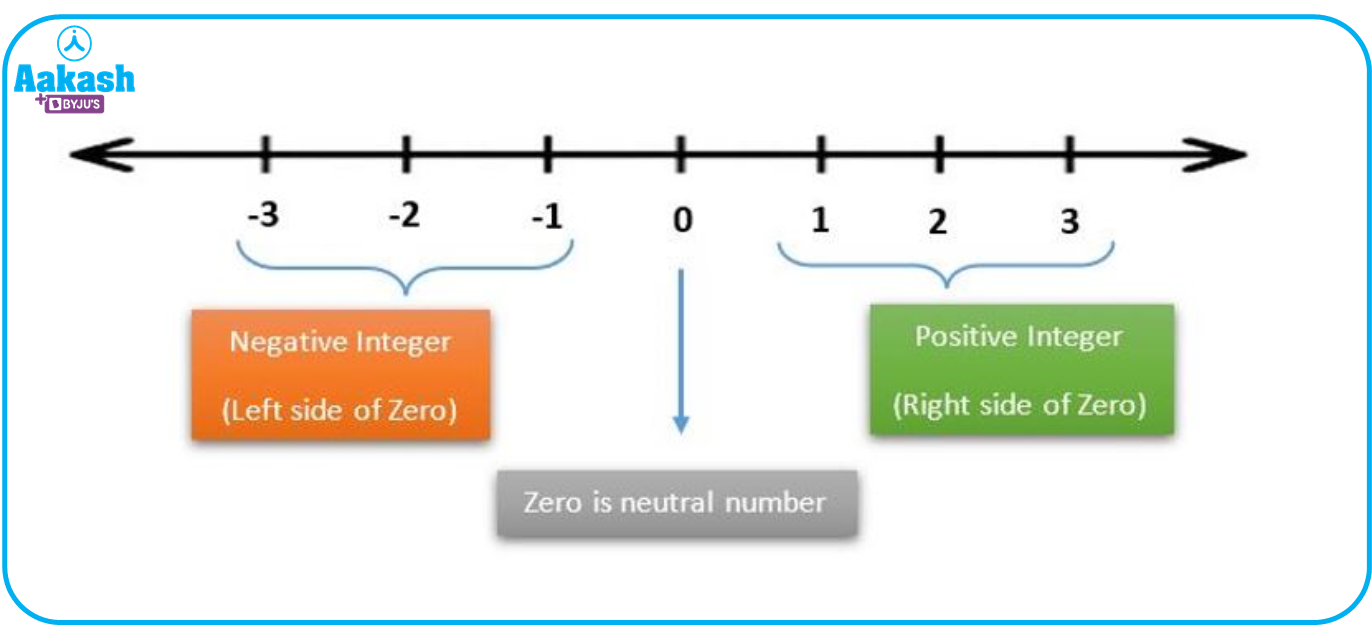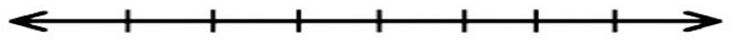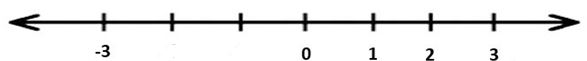• Call Now

1800-102-2727•

# Integer: Definition, Types, Operations, Properties, Practice Problems and FAQs

The term ‘Integer’ emerges from the Latin word ‘Integer’ meaning ‘Whole’ or ‘untouched’. In mathematics, an integer is a collection of counting numbers (Natural numbers) including zero and negative of counting numbers. We can make a statement for the integer that it can be zero, a positive number, or a negative number but it cannot be a fraction. In this article, we will learn about the definition of integers, types of integers, arithmetic operations like subtraction, addition, multiplication and division of integers, properties of integers and many solved examples. Now, let us discuss them all in detail.

## Definition of Integers

Integers is a collection of natural numbers including zero and negative of the natural numbers.

For example: ...,-3,-2,-1,0, 1, 2, 3,...

The collection of integers is represented by Z, where Z stands for Zahlen, which means to count.

## Types of Integers

Integers are of three types:

1. Positive Integers (Z+)
2. Negative Integers (Z-)
3. Zero (0)

## Positive Integers

Any number greater than zero is said to be a positive number and here if we are talking about positive integers so the counting numbers or natural numbers are the positive integers. It is denoted by ‘Z+’. The positive integers lie on the right side of zero on the number line.

For example:

Z+ = { 1, 2, 3,...,∞ }

## Negative Integers

Any number less than zero is said to be a negative number and if we are talking about negative integers so the negative of natural numbers is called negative integers. They are denoted by 'Z-'. The negative integers lie on the left side of zero on the number line.

For example

Z- = {-∞,...,-3,-2,-1}

## Zero

Zero (0) is neither a positive nor a negative integer. It is called a neutral number and there is a reason behind this because it has no sign i.e., '+' and '-'.

Note:- Negative integers are also called the additive inverse of positive integers.

## Graphical Representation of Integers on Number Line

Till this point, we have a basic idea about integers. Now, let us learn how to represent them on the number line so we can understand the role of integers in mathematics more clearly.

Before representing integers on a number line, let us understand what a number line is. Basically, the number line is the visual representation of numbers on a straight line in both directions (positive and negative) and zero will be at the centre of the number line.

On a number line, the positive integers will always be on the right side of zero and the negative integers will be on the left side of zero as shown in the figure below:Number Line

Note:

If there is no symbol of the operator in front of a number then the number is positive. i.e., 5 or +5 both are the same.

## Arithmetic Operations on Integers

The four basic operations performed on integers are-

2. Subtraction of integers
3. Multiplication of integers
4. Division of integers

Adding integers means an addition between two or more integers where the final result might increase or decrease depending on the sign of the integers.

• Adding integers of the same sign:

Adding the integers of the same sign means adding the numerical values and writing down the same sign provided with the numbers.

For example,

5 + 11=16

-5 +(- 11)=-16

• Adding integers of different signs:

While adding a positive and negative number, find the difference in the numerical value of the numbers and give the sign of the number having a larger numerical value.

For example,

(-6)+2=-4

(6)+(-2)=4

### Subtraction of Integers

When we subtract two integers, first we should change the sign of the second integer by which the first integer is being subtracted and apply the same rule of addition.

For example,

5 -2=5+(-2)=3

3-8=3+(-8)=(-5)

### Multiplication of Integers

There are some rules to be followed to carry out the multiplication of integers. The different rules and possible cases for the multiplication of integers are given below in the table.

 Signs Final result Example +$×$ + + + $×$- - -$×$ + - -$×$ - +

### Division of Integers

Division of integers means dividing an integer equally in a group or dividing into a specific number of groups. The rules of division are similar to that of multiplication.

In order to perform division, there are several rules given below in the table.

 Signs Final result Example (+) ÷ (+) + 6 ÷ 3=2 (+) ÷ (-) - 6 ÷ (-3)=(-2) (-) ÷ (+) - -6 ÷ 3=(-2) (-) ÷ (-) + (-6) ÷ (-3)=2

Note:

Division of integers is not defined by 0.

## Properties of Integers

The important properties followed by integers are as follows:

1. Closure Property
2. Commutative Property
3. Associative Property
4. Distributive Property

### Closure Property

If we perform any operation on any two integers and the outcome is also an integer then we can say that the integers are closed under those operations. Integers are closed under addition, subtraction and multiplication.

The closure property is applicable to the following operations when we have two integers (say), r and s;

For example,

2+3=5

5-2=3

Note: Integers are not closed under division. For example: 1 ÷ 2=0.5 which is not an integer.

### Commutative Property

According to the commutative property of integers, if r and s are the two integers, then:

• $r+s=s+r$

For example,

• $5+9=9+5=14$

Note: Commutative property is only applicable in case of addition and multiplication of integers.

### Associative Property

According to the associative property of integers, if r, s and t are integers, then:

• $r+\left(s+t\right)=\left(r+s\right)+t$

For example,

• $1+\left(3+5\right)=\left(1+3\right)+5=9$

Note: Associative property is only applicable in case of addition and multiplication of integers.

### Distributive Property

Integers follow the distributive property over addition and subtraction as shown and $r×\left(s-t\right)$, it means operand r can be distributed among the operands s and t as,

• $r×\left(s-t\right)=\left(r×s\right)-\left(r×t\right)$

For example,

On adding any number to a given number if the result is zero then that number is the additive inverse of the given number.

Here, (-r) is the additive inverse of r.

For example,

• 2+(-2)=0

### Multiplicative Inverse

On multiplying a number with a given number if the final result is one then that number is the multiplicative inverse of the given number. Generally, the reciprocal of a number is the multiplicative inverse of the original number.

Here, the multiplicative inverse of an r is $\frac{1}{r}$.

For example,

Note:- Zero doesn’t have a multiplicative inverse.

On adding any number to a given number if the result remains the same then that number is the additive identity of the given number. i.e,$r+0=r=0+r$

For example,

8+0=8=0+8

### Multiplicative Identity

On multiplying a number with a given number if the final result remains the same, then that number is the multiplicative identity of the given number.

i.e.,

One is the multiplicative identity.

For example,

Absolute Value of an Integer

The absolute value of an integer is its numerical value irrespective of sign and it is denoted by two vertical bars i.e. | |

Example: |-5|=5 and |+5|=5

## Practice Problems

1. Solve the following:
• 5 +8 =
• (-8)+7 =
• (-25) +(-14) =
• 4 -19 =

Solution:

• 5 +8 = 13
• (-8)+7 =(-1)
• (-25) +(-14) =(-39)
• 4 -19 =(-15)
1. Solve the following:

Solution:

1. Plot the following integer on the given number line:

3,-3, 1, 2Solution:1. Solve the division of integers:
• 15 ÷ 3 =
• 39 ÷ -13 =
• -75 ÷ -5 =

Solution:

• 15 ÷ 3 =5
• 39 ÷ -13 = -3
• -75 ÷ -5 =15

## FAQs

Q1. How many integers will lie between 0 and 1?
There is no integer lying between 0 and 1 as 0 and 1 are consecutive integers.

Q2. What are consecutive integers?
It is a sequence of integers for which the common difference is 1.

For example: 4 and 6 are the consecutive integers for integer 5.

Q3. What are the types of integers?
There are three types of integers i.e, positive integers, negative integers and zero.

Q4. What is the difference between additive inverse and multiplicative inverse?